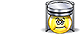Unit Conversion Software Web Widgets Loan Calculator Currency Rates Country Flags Unit Converter
 Faq Help# Standard Atmosphere ConversionContact

## Standard Atmosphere, unit of measure

Standard atmosphere (symbol: atm) is a unit of pressure. Standard atmosphere is a non-SI unit that is internationally recognized. The 10th Conférence Générale des Poids et Mesures (10th CGPM) adopted standard atmosphere for general use and affirmed its definition of being precisely equal to 1,013,250 dynes per cm^2 (101,325 Pa). This value is intended to represent the mean atmospheric pressure at mean sea level at the latitude of Paris, France, and as a practical matter, truly reflects the mean sea level pressure for many of the industrialized nations (those with latitudes similar to Paris).
To perform conversions between Standard atmosphere and other Pressure units please try our Pressure Unit Converter

Convert Standard atmosphere to:
pascal, exapascal, petapascal, terapascal, gigapascal, megapascal, kilopascal, hectopascal, dekapascal, decipascal, centipascal, millipascal, micropascal, nanopascal, picopascal, femtopascal, attopascal, newton/square meter, newton/square centimeter, newton/square millimeter, kilonewton/square meter, bar, millibar, microbar, dyne/square centimeter, kilogram-force/square meter, kilogram-force/square centimeter, kilogram-force/square millimeter, gram-force/square centimeter, ton-force (short)/square foot, ton-force (short)/square inch, ton-force (long)/square foot, ton-force (long)/square inch, kip-force/square inch, ksi, pound-force/square foot, pound-force/square inch, psi, poundal/square foot, torr, centimeter mercury (0°C), millimeter mercury (0°C), inch mercury (32°F), inch mercury (60°F), centimeter water (4°C), millimeter water (4°C), inch water (4°C), foot water (4°C), inch water (60°F), foot water (60°F), atmosphere technicalDownload Pressure Unit Converter
our powerful software utility that helps you make easy conversion between more than 2,100 various units of measure in more than 70 categories. Discover a universal assistant for all of your unit conversion needs - download the free demo version right away!Copyright © 1998-2009 UnitConversion.org Privacy & Terms | About | Faq | Help | Contact | Link to Us